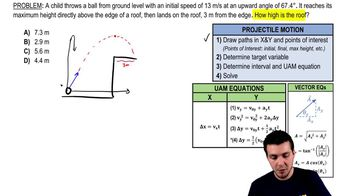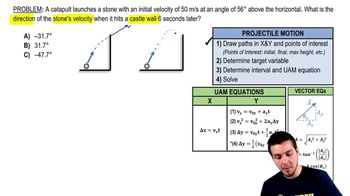Start typing, then use the up and down arrows to select an option from the list.
9:27 minutes
Problem 3k
Textbook Question

# In a carnival booth, you can win a stuffed giraffe if you toss a quarter into a small dish. The dish is on a shelf above the point where the quarter leaves your hand and is a horizontal distance of 2.1 m from this point (Fig. E3.19). If you toss the coin with a velocity of 6.4 m/s at an angle of 60° above the horizontal, the coin will land in the dish. Ignore air resistance. (a) What is the height of the shelf above the point where the quarter leaves your hand? (image)Verified Solution
This video solution was recommended by our tutors as helpful for the problem above.
62views